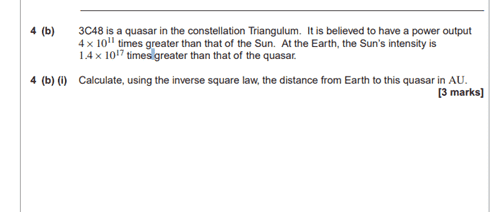# Intensity and power question (weird)https://pmt.physicsandmathstutor.co...e 2016 MS - Unit 5-2A AQA Physics A-level.pdf

One question on this the mark scheme seems to skip this step as the intensity is Power per unit area ,
when you make I sun = I quaser * 4*10^17 and you plug in the values for power per unit area would the 4 pies cancel each other out
so P/4pieD^2 = ( P*4*10^11/4pieD^2 ) * 4* 10^12 ?
one final question on this when using the intensity equation should i assume the distance from a point to the emitting source of radiation affected by the inverse square law is a giant circle essentially ? As they want the distance from earth to the quaser would that mean that the P/ 4pie D^2 value the D value is the distance of the two objects not the surface area of the star or object ?

Doc Al
Mentor
Treat the sun and quasar as point sources. Then realize that the intensity follows the inverse square law, so ##I \propto \frac{P}{r^2}##.

•bonbon22
Treat the sun and quasar as point sources. Then realize that the intensity follows the inverse square law, so ##I \propto \frac{P}{r^2}##.
I seee thats interesting Doc, in most astronomical distances should i always treat the suns and quasers as point sources unless the question states otherwise?
and also Is P/r^2 not equal to the intensity if thats the case and the 4 pie no longer applies so it would be = to and not proportional to intensity ?

Doc Al
Mentor
in most astronomical distances should i always treat the suns and quasers as point sources unless the question states otherwise?
I would.
and also Is P/r^2 not equal to the intensity if thats the case and the 4 pie no longer applies so it would be = to and not proportional to intensity ?
Since all you care about are ratios, the 4π drops out. But you're correct: ##I = \frac{P}{4\pi r^2}##.

•bonbon22
I would.

Since all you care about are ratios, the 4π drops out. But you're correct: ##I = \frac{P}{4\pi r^2}##.
one final question Doc if a question was not based on " ratios" does the 4 pie drop out as it is a point source?

Doc Al
Mentor
one final question Doc if a question was not based on " ratios" does the 4 pie drop out as it is a point source?
Not quite sure what you mean. If you are given the power and asked to find the intensity at some distance from the point source, then you'd need the full equation with the 4π included.

•bonbon22
Not quite sure what you mean. If you are given the power and asked to find the intensity at some distance from the point source, then you'd need the full equation with the 4π included.
okay got you, one very final question to clear my mind , should i assume that the D value is the diameter of a very large circle which essentially touches the earth. as intensity is power / surface area <----- and this surface area is just a really big circle.

Doc Al
Mentor
okay got you, one very final question to clear my mind , should i assume that the D value is the diameter of a very large circle which essentially touches the earth. as intensity is power / surface area <----- and this surface area is just a really big circle.
Treat the distance as the radius of a large sphere, whose total area is ##4\pi D^2##. (Where D is the distance from the source to the earth.)

•bonbon22